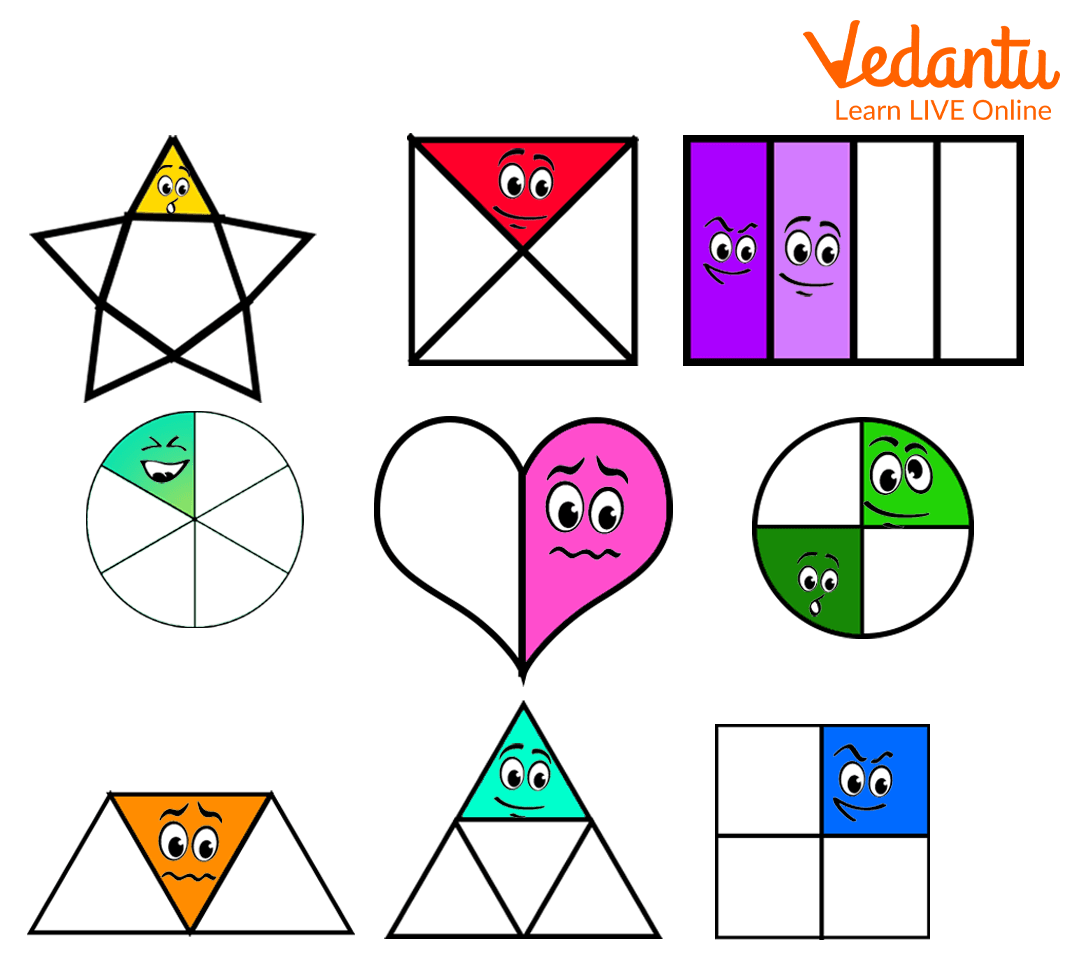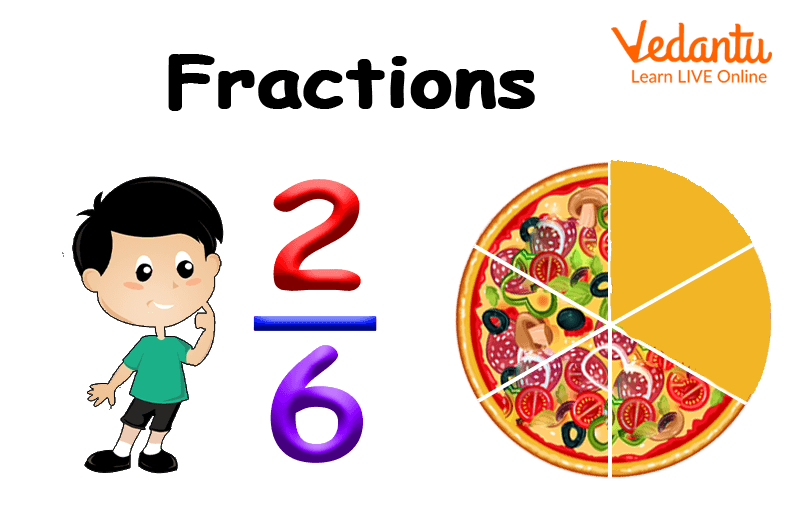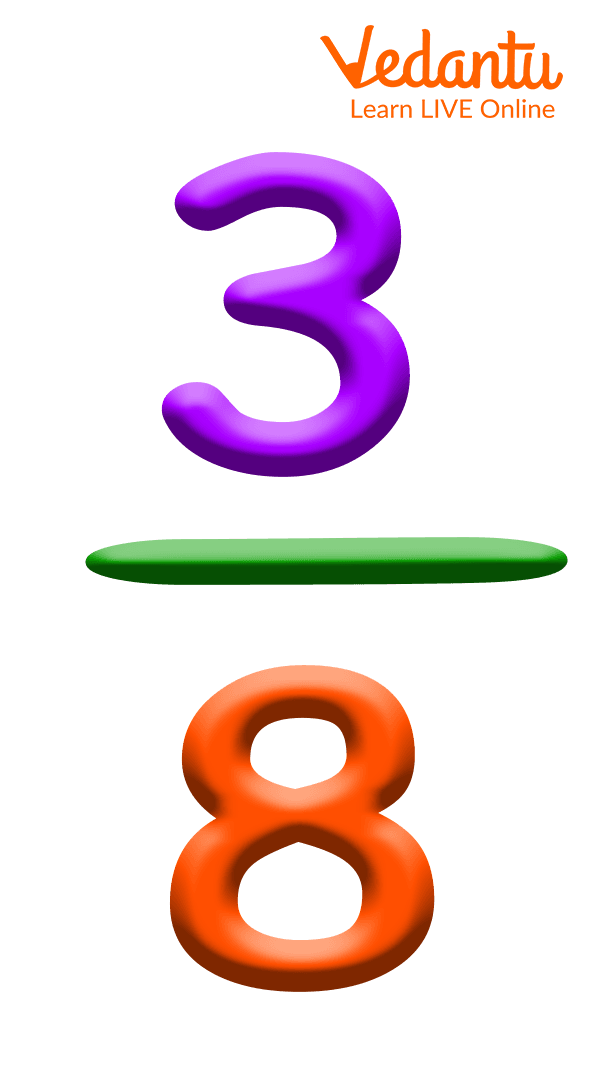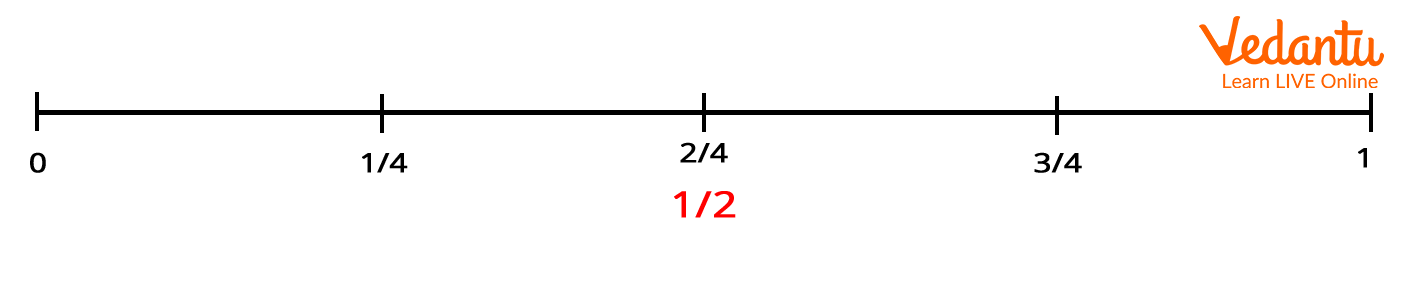Courses
Courses for Kids
Free study material
Free LIVE classes
More

# Exploring Fractions in Year 2 (For Kids of Age 6-7 years)LIVE
Join Vedantu’s FREE Mastercalss

## What Are Fractions?

In Year 2 Maths, kids generally learn to identify fractions and recognise that they are different from the whole numbers. By Year 2, kids become well aware of the whole numbers, it is the right time to introduce the concept of halves, thirds, and quarters to them. Understanding fractions can be easier when explained with various pictorial representations. Two of the fundamental concepts of fractions comprise simplification of fractions and recognising the equivalence of fractions, and there are several ways to explain these concepts to your kids.

So here we have put together some of the best ways to introduce the concepts of fractions in Year 2.

## Introducing Fractions with Fun Crafts

Often kids tend to be reluctant when it comes to their same study routine. So we can take a little detour from the Maths book and worksheets to the colourful stationery of drawing and craft to introduce the concept of fractions to them. Open the box of colourful papers, craft scissors, colour pencils, and pens to make some common geometric shapes like squares, circles, etc. Ask your kids to fold the shapes into two equal parts to explain the halves.Folded paper shapes

Similarly, ask them to fold the shapes into three, four, or more equal parts and each time they fold, tell them about the fractions hence achieved. Also, to make it even more insightful, you can ask them to identify the smaller shapes obtained by folding the original shapes of paper.

## Explaining Fractions with Real-life Objects

Real-life objects like pizzas, watermelons, etc. can be of great help when it comes to explaining to kids, ‘what are fractions?’. Any object that they are familiar with or maybe fond of will definitely grab their attention in this case. For example, when you take your kids out on a pizza treat, ask them to count the number of slices in the whole pizza.Fractions of a pizza

Bring it to their attention that the pizza is divided into equal slices, and then while doling out the slices on their plates, explain the fraction of pizza they are having. You may try the same with a home-baked cake. When you bake your kid’s favourite cake, cut it into as many equal pieces as you wish. With each piece of cake you serve your kid, tell them the fraction of cake involved.

Try explaining the concept of fractions with as many real-life objects as you wish as it will provide your kid with a clear understanding of fractions.

## Writing Down the Numerators and Denominators

Once your child is aware of the idea of halves, thirds, and fourths, it is time for them to learn to write these fractions. The basic concept of numerators and denominators will be new for kids learning fractions in Year 2. So, you may use big and colourful text, preferably with cartoons, to introduce the numerators and denominators at first.A fraction

This will set a clear picture of the parts of a fraction for kids. They will be able to identify the part of the fraction written above the bar as the numerator and that written below the bar as the denominator. The denominator tells us the total number of equal parts given, whereas the numerator tells us the number of equal parts we consider of them all.

## Colour-Blocking Activities

To explain how to decode a given fraction, you can make your kids do various colour-blocking activities. For example, draw three squares and divide the first one into two equal parts, the second one into three equal parts, and the third one into four equal parts. Now write some random fractions like, 1/2, 2/3,3/4 etc., and ask your child to fill the parts represented by the numerators, with their favourite colour, in the corresponding squares.

## Recognising Equivalent Fractions

Once your child is able to colour the parts correctly, it is time to introduce the concept of equivalent fractions. Write some more random fractions that are equivalent to the fractions you wrote previously. Draw a new square and divide it into four equal parts. Write an equivalent fraction, say, 24 and let your child find if there is any common factor that can divide both the numerator and the denominator. Input ideas to think about the multiple tables to stimulate the thinking process. Create more of such activities to explain the concept of equivalent fractions clearly.

## Fractions on the Number Line

After getting a clear understanding of the numerator and denominator of fractions, the time is ripe to introduce the concept of number line. This will help them understand that fractions exist between whole numbers. To retain your child’s attention, draw the number line with their favourite colour pen.Fractions on the numbers line

Mark any two numbers, it is preferable to begin with 0 and 1, as these are the very first numbers any child learns. Start marking with 1/2 right at the mid-way between 0 and 1, explain the count from 0 to 1/2 and from 1/2 to 1. Similarly, go on marking more fractions between the interval of 0 and 1. Remember explaining the count each time you mark a new fraction.

## Conclusion

It is always quite fruitful to introduce and explain any concept to kids with the help of objects and things they are familiar with. The use of colours, crafts, and animated images can also be helpful to grab their attention while explaining new ideas. The above-listed ways are only a few ideas to introduce the concept of fractions to kids. You can also try giving them other objects like toys, fruits, cookies, etc. and group the objects equally to explain fractions to your kids. Check out the interesting ideas available on Vedantu to introduce new concepts of maths to young kids for a hassle-free learning process.

Last updated date: 03rd Oct 2023
Total views: 137.7k
Views today: 3.37k

## FAQs on Exploring Fractions in Year 2 (For Kids of Age 6-7 years)

1. What are halves and quarters?

The most fundamental concept of fractions are halves, thirds, or quarters. To obtain a half of something, we divide it by 2. To obtain the quarter of something, we divide it by 4. Therefore, to represent one-half, 1/2, we write 1 in the numerator and 2 in the denominator of a fraction. Similarly, to represent one-quarter, 1/4 ,we write 1 in the numerator and 4 in the denominator of a fraction.

2. What are equivalent fractions?

When two or more fractions have different denominators but on simplification they yield the same value, such fractions are referred to as equivalent fractions. For example,1/2,2/4,4/8 are equivalent fractions as they yield the same value 1/2 on simplification.